Ch.13 - Chemical KineticsWorksheetSee all chapters
 Ch.1 - Intro to General Chemistry 4hrs & 4mins 0% complete Worksheet Ch.2 - Atoms & Elements 4hrs & 21mins 0% complete Worksheet Ch.3 - Chemical Reactions 4hrs & 18mins 0% complete Worksheet BONUS: Lab Techniques and Procedures 1hr & 38mins 0% complete Worksheet BONUS: Mathematical Operations and Functions 47mins 0% complete Worksheet Ch.4 - Chemical Quantities & Aqueous Reactions 3hrs & 54mins 0% complete Worksheet Ch.5 - Gases 3hrs & 22mins 0% complete Worksheet Ch.6 - Thermochemistry 2hrs & 26mins 0% complete Worksheet Ch.7 - Quantum Mechanics 2hrs & 17mins 0% complete Worksheet Ch.8 - Periodic Properties of the Elements 2hrs & 43mins 0% complete Worksheet Ch.9 - Bonding & Molecular Structure 2hrs & 52mins 0% complete Worksheet Ch.10 - Molecular Shapes & Valence Bond Theory 1hr & 31mins 0% complete Worksheet Ch.11 - Liquids, Solids & Intermolecular Forces 3hrs & 40mins 0% complete Worksheet Ch.12 - Solutions 2hrs & 17mins 0% complete Worksheet Ch.13 - Chemical Kinetics 2hrs & 23mins 0% complete Worksheet Ch.14 - Chemical Equilibrium 2hrs & 26mins 0% complete Worksheet Ch.15 - Acid and Base Equilibrium 4hrs & 42mins 0% complete Worksheet Ch.16 - Aqueous Equilibrium 3hrs & 48mins 0% complete Worksheet Ch. 17 - Chemical Thermodynamics 1hr & 44mins 0% complete Worksheet Ch.18 - Electrochemistry 3hrs & 3mins 0% complete Worksheet Ch.19 - Nuclear Chemistry 1hr & 31mins 0% complete Worksheet Ch.20 - Organic Chemistry 3hrs 0% complete Worksheet Ch.22 - Chemistry of the Nonmetals 2hrs & 1min 0% complete Worksheet Ch.23 - Transition Metals and Coordination Compounds 1hr & 54mins 0% complete Worksheet

# Rate Law

See all sections
Sections
Rate of Reaction
Average Rate of Reaction
Arrhenius Equation
Rate Law
Integrated Rate Law
Collision Theory

Rate Law represents an equation for a chemical reaction that connects the reaction rate with the concentrations or pressures of the reactants and the rate constant.

###### Rate Law

When it comes to the rate of a reaction the Rate Law focuses on the reactant concentrations, while ignoring the product concentrations.

Concept #1: Understanding the Rate Law.

Transcript

Hey, guys, in this brand new video, we’re going to take a look at the new topic, rate law.
Now up to this point, we should know what general rate is. General rate is just the change in the concentration or amount of a compound, whether be a product or a reactant, over time. Now we are talking about rate law. Rate law is very different than general rate. For one, rate law does not care about the amounts of the product. It only focuses on the reactants. So that’s the biggest thing you need to worry about here, cares only about the reactants.
Remember I told us earlier that sometimes our reactions go to completion, they have a solid arrow going towards products, but sometimes our chemical reactions may have double arrows, meaning they’re at equilibrium. That means our reaction goes in the forward and the reverse direction.
We’re going to say that although these chemical reactions can be reversible, meaning they can go either way, we will only look at the forward reactions so we’re only looking at the forward direction for now. And because we are looking at only the forward direction that means we’ll be ignoring the reverse direction. So we’re ignoring how it goes backwards.
And if we’re only looking at the forward direction, then the rate is only dependent on reactant concentrations because as we’re going forward what can affect our speed of our reaction is how much reactants do I have to begin with. How long is it going to take me to break down all of those reactants to make my products?
And not only the reactant concentration, but were also going to focus on a new variable, k. Now we’re going to say that rate law is equal to k. Then we’re going to have the concentration of just the reactants. So as you can see rate law only cares about the reactants the product is nowhere to be found within our equation.
Then we’re going to say NO is to the x, O2 is to the y, these are also new variables we have to familiarize ourselves with. Here we going to say k is called our rate constant, and then we’re going to say x and y are called our reaction orders.

By focusing on only the reactant concentrations we only care about the forward direction of our chemical reaction.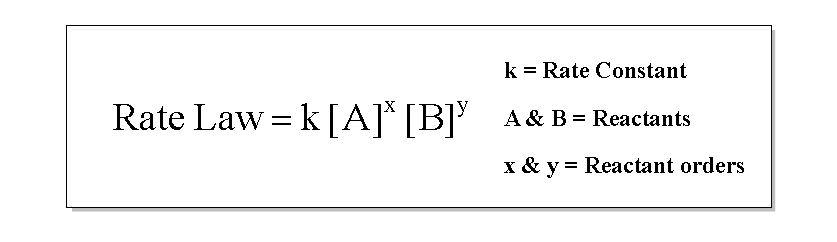Concept #2: The Rate Determining Step.

Transcript

This is what we need to remember here. We're going to say unless the reaction, the reaction has to say this. Unless the reaction is classified as a slow step, and the slow step is the rate determining step, then x and y one must be calculated experimentally.
If we take a look at the balanced equation, we have 4 moles of NO, 1 mole of O2. Just because those are the coefficient does not mean that x here is 4 and y here is 1. The only time that would be true is if your professor tells you explicitly that it's a slow reaction. If they don't tell you it’s slow, you cannot do this. I never told us the reaction is slow, so we would have to find that x and y experimentally.
Later on we'll see what exactly does that mean, and what kind of work do we need to do to find x and y. But just remember, for now it has to say slow step for you to just use the coefficients.

If the reaction says it’s a SLOW step or a ONE-STEP MECHANISM then we can simply look at the coefficients of the reactants to determine the reaction orders.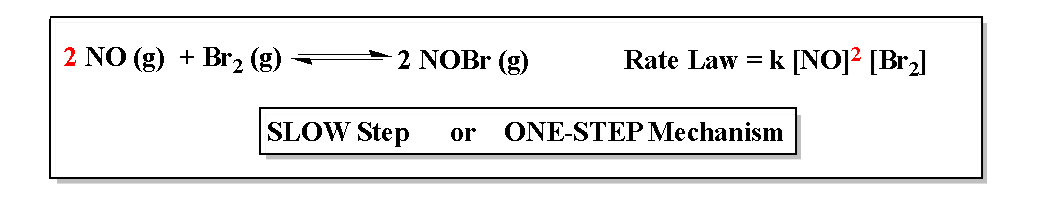Example #1: For the following reaction, use the given rate law to determine the best answer for the reaction with respect to each reactant and the overall order.

H2O2 (aq) + 3 I (aq) + 2 H+ (aq)  --->  I3 + 2 H2O (l)

Rate = k [H2O2]2 [I ]

a) H2O2 is 1st order, I is 1st order, 2nd order overall.

b) H2O2 is 2nd order, I is 1st order, 3nd order overall.

c) H2O2 is 0th order, I is 1st order, H+ is 1st order, 3rd order overall.

d) H2O2 is 2nd order, I is 1st order, H+ is 0th order, 3rd order overall.

Example #2: Answer each of the following question based on the following chemical reaction:

Calculate the reaction order for reactant A.

Practice: Calculate the reaction orders for Reactants B and C.

Example #3: Calculate the rate constant and the new rate for the given reaction if the initial concentration of [A] = 0.300 M, [B] = 0.150 M and [C] = 0.150 M.

###### The Rate Constant k

The Rate Constant k can have a direct impact on the rate of the reaction. In order to determine its units just utilize the equation below, where n is the overall order of the reaction: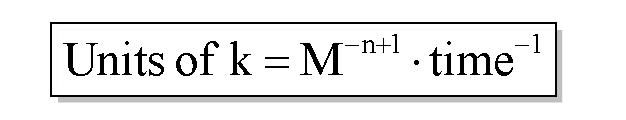Example #4: A certain chemical reaction has the given rate law:

Rate = k [A]3 [B]2 [C]-1

What are the units of the rate constant for the given reaction?

a) M-2 s-1      b) M2 s-1       c) M-4 s-1      d) M-3 s-1      e) M3 s-1

Transcript

Hey guys! In this new video, we're going to take a closer look at rate and look at the calculations involved. We’d figure out the units for the rate constant k.
Let's take a look at the first question. It says: A certain chemical reaction has the given rate law: Rate equals k A to the 3, B to the 2, and C to the negative 1. What are units of the rate constant for the given reaction? They're asking us to find the units for rate constant k. All you need to realize here is that when it comes to figuring out the units for k, just use the simple equation: k equals the molarity to the negative n plus 1, times time inverse.
Remember, we talked about the variable n earlier. We said n equals the overall order. How do we find out the overall order of a reaction? We just added up all of the reaction orders. Here 3 plus 2 plus negative 1. When we do that, we get n equals 4, so n here equals four. We're going to plug that in. M to the negative 4 plus 1, times here all the units of time are in seconds inverse so we'll just use times second inverse. Remember, time could be in minutes, in seconds, hours. It doesn't really matter. Then we're going to say here negative 4 plus 1 is negative 3. Here the correct answer would be m to the negative 3 times seconds to the negative 1. The correct answer would be D.
Remember, this is really saying M to the inverse three. What does that really mean? We could have also written the answer as this. Inverse means that it's really 1 over M to the third and then seconds is inverse too, so that's 1 over s. Those two are multiplying so it’d really be 1 over M to the third times seconds. Remember, this is just basic algebra. Inverse really means one over whatever that power is. If I gave you something to the inverse half, then that really means 1 over A to the half. Remember if you're the half power, that's another way of saying square root.
We’ll take a look at more of how do we manipulate inverse functions later on down this page.

Example #5: The reaction of 3 A + B ---> 2 C + D, was found to be: Rate = k [A]2 [B]3. How much would the rate increase by if A were tripled while B were increased by half?

a) 0.50              b) 30.38            c) 0.75              d) 20.25            e) 1.125

Practice: If the rate law for the following reaction is found to be the following, what are the units for the rate constant, K?

Cl2 (g) + HCCl3 (g) → HCl (g) + CCl4 (g)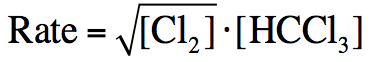a)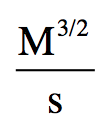b)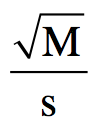c)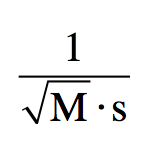d)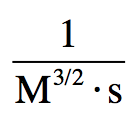e)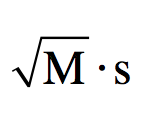###### Reaction Mechanisms

Concept #3: A   Reaction Mechanism   involves a series of elementary reactions that give the overall equation.

Transcript